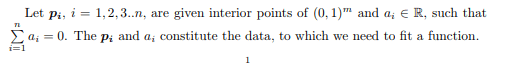# #StackBounty: #machine-learning A maximization problem, with motivation in machine learning

### Bounty: 50

Consider the minimization problem described this paper. Let $$f_{lambda}$$ be the minimizer. As a part of extending my work, I am able to show the following facts

$$lim_limits{lambda to 0}|f_{lambda}|{L^2} = 0$$ and $$lim_limits{lambda to infty}|f$${lambda}|_{L^2} = 0\$\$

My problem now is (as I would like to extend my work), find $$lambda in (0,infty)$$ for which $$|f_{lambda}|_{L^2}$$ is maximum. Appreciate your suggestions to solve this problem.

The minimization problem from the linked paper is given below for the self containment of the post. If given that $$k>frac{m}{2}$$, the paper proves that there is a unique minimizer for the functional $$C(f)$$ in the set $$S$$.It is given that $$k>frac{m}{2}$$

## My progress

Partial Progress :

Progress : I am able to derive the corresponding PDE equations for the problem.

Let $$f(lambda,x) = f_{lambda}(x)$$. ThenThe first equation corresponds to maximizing $$|f_{lambda}|$$, while the second PDE is for the minimization problem associated with the parameter $$lambda$$.

The second equation (minimization problem), given any $$lambda$$, I can solve for $$f(lambda,.)$$ either using linear algebra or steepest descent algorithm, which I have described in my article. Now I need to use this solution and the first equation to obtain $$lambda$$, which is a problem I am facing.

Trying to solve using linear algebra, by formulating the discrete version of the problem using Fourier series coefficients and Plancheral theorem, I get stuck at the matrix problem described here.

More Partial Progress

An Iterative algorithm which is a modified steepest descent.

1. Initialize $$f$$.

2. Assuming some $$lambda$$ and assuming gradient of $$C_{lambda}(f)$$ wrt $$f$$ be $$nabla_f C_{lambda}(f)$$, and if we were to update $$f$$
with this gradient as in we do in steepest descent, it would be
$$f^u_lambda = f – delta nabla_f C_{lambda}(f)$$, where $$delta$$ is
a constant learning rate. Now set
$$frac{partial|f^u_lambda|}{partial lambda} = 0$$ and solve for
$$lambda$$. Let the root be $$lambda_0$$.

3. Update $$f = f^u_{lambda_0}$$. (update $$f$$ as in steepest descent, but using $$lambda$$ value as $$lambda_0$$ which was computed in step
2.)
4. check some convergence criterion and if not met, go to step 2.

I have implemented this numerically and it converges as desired. Need to work on the proof.

PS : This was first posted on MO by me, 3 months back. Link and cross posted on math.SE here.

Get this bounty!!!

This site uses Akismet to reduce spam. Learn how your comment data is processed.